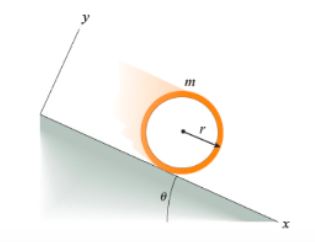# Problem: Part A. What is a, the acceleration of the hoop’s center?Express your answer in terms of some of all of the variables m, θ, and g, the acceleration due to gravity.Part B. What is the minimum coefficient of static friction, µmin, needed for the hoop to roll without slipping? Note that it is static and not kinetic friction that is relevant here, because the bottom point on the hoop is not moving relative to the ground (which is what it is meant by no slippage).Express your answer in terms of all of some of the variables m, r, and θ

###### FREE Expert Solution

The moment of inertia of a hoop about its center is:

$\overline{){{\mathbf{I}}}_{\mathbf{c}\mathbf{m}}{\mathbf{=}}{\mathbf{m}}{{\mathbf{r}}}^{{\mathbf{2}}}}$

Part A.

Let's consider the equation of motion along the x-axis:

mgsinθ - T = ma ..................... (1) where a is the acceleration down the incline.

Rotational motion equation:

88% (163 ratings)###### Problem DetailsPart A. What is a, the acceleration of the hoop’s center?
Express your answer in terms of some of all of the variables m, θ, and g, the acceleration due to gravity.

Part B. What is the minimum coefficient of static friction, µmin, needed for the hoop to roll without slipping? Note that it is static and not kinetic friction that is relevant here, because the bottom point on the hoop is not moving relative to the ground (which is what it is meant by no slippage).
Express your answer in terms of all of some of the variables m, r, and θ